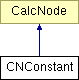# CNConstant Class Reference

Calculation node always returning a constant value. More...

`#include <expression.h>`

Inheritance diagram for CNConstant:List of all members.

## Public Member Functions

CNConstant (double _value)
construct a constant calculation node from a value
virtual double evaluate () const
evaluate the complete calculation tree and return the floating point result value
virtual void print (std::ostream &os, unsigned int depth) const
output the calculation tree to the given stream.

## Private Attributes

double value
the constant value returned

## Detailed Description

Calculation node always returning a constant value.

Definition at line 39 of file expression.h.

## Constructor & Destructor Documentation

 CNConstant::CNConstant ( double _value ) ` [inline, explicit]`

construct a constant calculation node from a value

Definition at line 46 of file expression.h.

## Member Function Documentation

 virtual double CNConstant::evaluate ( ) const` [inline, virtual]`

evaluate the complete calculation tree and return the floating point result value

Implements CalcNode.

Definition at line 51 of file expression.h.

References value.

 virtual void CNConstant::print ( std::ostream & os, unsigned int depth ) const` [inline, virtual]`

output the calculation tree to the given stream.

tries to format the output to make tree levels visible.

Implements CalcNode.

Definition at line 56 of file expression.h.

References CalcNode::indent(), and value.

## Member Data Documentation

 double CNConstant::value` [private]`

the constant value returned

Definition at line 42 of file expression.h.

Referenced by evaluate(), and print().

The documentation for this class was generated from the following file:

Generated on Sun Aug 3 12:34:09 2008 for Flex Bison C++ Example by1.5.5